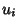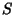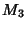## Stäckel Determinant

A Determinant used to determine in which coordinate systems the Helmholtz Differential Equation is separable (Morse and Feshbach 1953). A determinant(1)

in whichare functions ofalone is called a Stäckel determinant. A coordinate system is separable if it obeys the Robertson Condition, namely that the Scale Factorsin the Laplacian(2)

can be rewritten in terms of functionsdefined by(3)

such thatcan be written(4)

When this is true, the separated equations are of the form(5)

Thes obey the minor equations(6)(7)(8)

which are equivalent to(9)(10)(11)

This gives a total of four equations in nine unknowns. Morse and Feshbach (1953, pp. 655-666) give not only the Stäckel determinants for common coordinate systems, but also the elements of the determinant (although it is not clear how these are derived).

See also Helmholtz Differential Equation, Laplace's Equation, Poisson's Equation, Robertson Condition, Separation of Variables

References

Morse, P. M. and Feshbach, H. Tables of Separable Coordinates in Three Dimensions.'' Methods of Theoretical Physics, Part I. New York: McGraw-Hill, pp. 509-511 and 655-666, 1953.